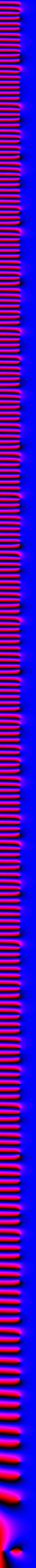# A Gallery of Complex Functions

### by François Labelle

 On these pages, you will see maps of the argument (also called phase) of some complex functions. For clarity and aesthetic reasons, I chose to disregard the magnitude completely. There are other galleries of complex functions on the Internet that will show both information. The color assignment is : 1 -> blue, i -> magenta, -1 -> red, and -i -> black. When the four colors are touching at a point, it means that the point is a zero or a pole (infinity). The resolution is of 5 pixels per unit. The range of every image is from -30 to 30 for both the real and the complex axes, except for the band on the left which is the Riemann zeta function from -5 to 5 and from -5*i to 435*i.

## Page 1: Miscellaneous functionsf(z) = z. This shows which color gets assigned to each complex number. The center is a good example of what a zero looks like.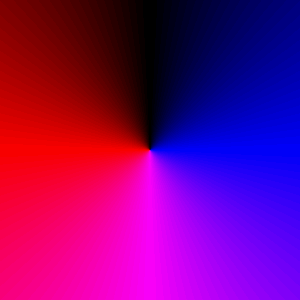f(z) = 1/z. The center is a good example of what a pole looks like. You can distinguish between a zero and a pole by the order in which colors appear as you go around counterclockwise.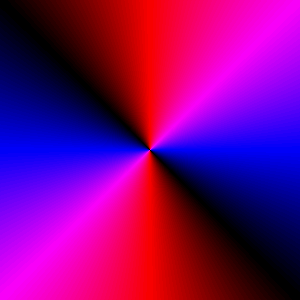f(z) = z^2. Recall: 1 or -1 squared give 1 (blue), i or -i squared give -1 (red).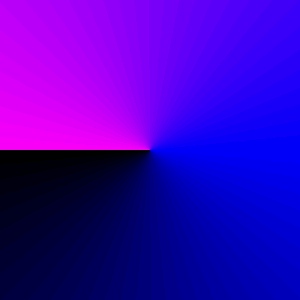f(z) = sqrt(z). As in the real case, we need to make a choice between two possible square roots. Whatever the choice, there will be at least one discontinuity, which is usually put pointing toward the left.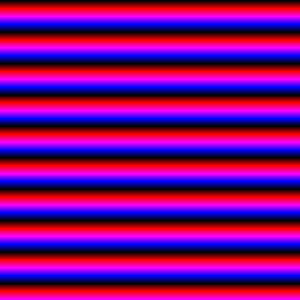f(z) = exp(z). The exponential growth cannot be seen because this is a phase diagram. The phase is cyclic along the imaginary axis with period 2*pi.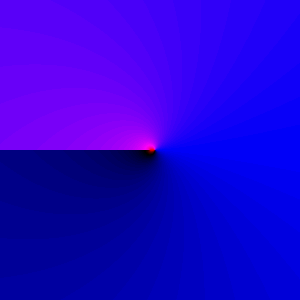f(z) = log(z). Recall that for 0

page last updated: March 16, 2002